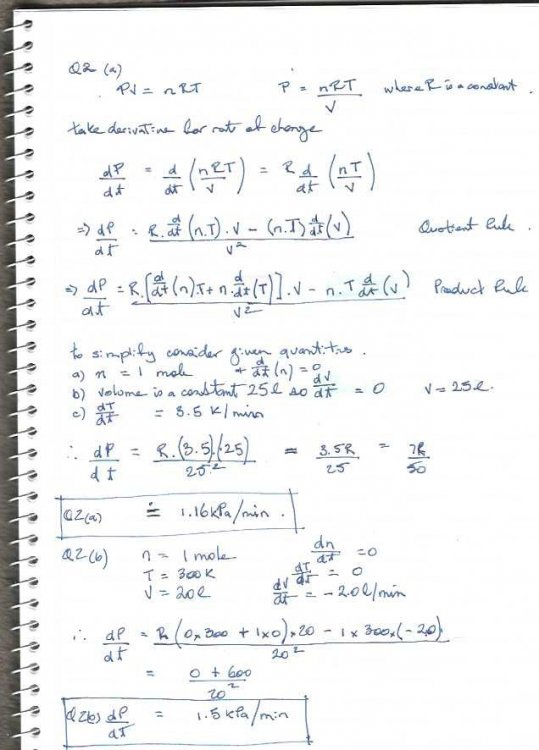# Change of rates problem - ideal gas law

## Recommended Posts

Hopefully during this period of isolation for many of us, someone can find time to check this. Some introductory remarks, this is a maths subject not physics and they really don't care about units but they do care that you simplify the answer as an accurate expression before approximating with the calculator. I have struggled with the differentiation so would appreciate thoughts there. This is just the first part at this stage.

QUESTION:

The ideal gas law relates the temperature, pressure and volume of an ideal gas. For n moles of gas the pressure P,  volume V, and temperature T are related by the equation:

PV = nRT

Where n is the ideal gas constant. If pressure is measured in kilopascals (kPa), volume in litres (L) and temperature in degrees kelvin (K) then R = 8.3145 kPaL/Kmol.

a) Suppose that one mole of ideal gas is held in a closed container with a volume of 25 litres. If the temperature of the gas is increased at a rate of 3.5 kelvin/min, how quickly will the pressure increase?

b) Suppose that the temperature of one mole of gas is held fixed at 300K, while the volume decreases at a rate of 2.0 litres/min. How quickly is the pressure of the gas increassing at the instant that the volume is 20 litres?

Edit: updated with proposed answers to both parts of the Q.Edited by druS
##### Share on other sites

8 hours ago, druS said:

The ideal gas law relates the temperature, pressure and volume of an ideal gas. For n moles of gas the pressure P,  volume V, and temperature T are related by the equation:

PV = nRT

Where n is the ideal gas constant. If pressure is measured in kilopascals (kPa), volume in litres (L) and temperature in degrees kelvin (K) then R = 8.3145 kPaL/Kmol.

n isn’t the ideal gas constant. It’s the number of moles of the gas in the sample (kilomoles, to be consistent with your units)

##### Share on other sites

• 2 weeks later...

Thanks Swansont, it was what i thought I was looking for but turns out you knew better than me.

Appreciated.

Dru

## Create an account

Register a new account